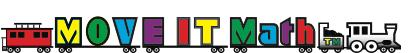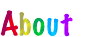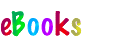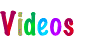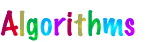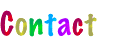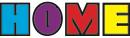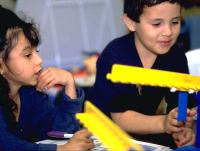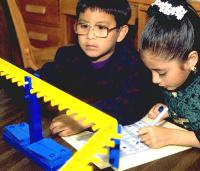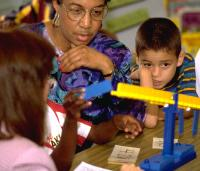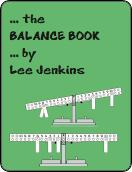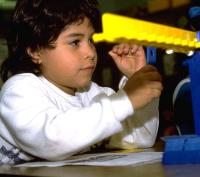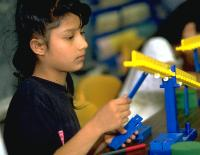The main cause of confusion about the equal sign in grade school math is the way it is presented in every textbook-based elementary school math curriculum in America today. Overwhelmingly, it is used to separate a problem from its answer with the answer appearing to the right of the problem. This does not mean that we should do away with math textbooks and math worksheets. What is needed is teacher or parental intervention to counter the incorrect mindset that is currently being promoted, however inadvertently. The following are strategies for doing so.

Model “equals” as “balanced” on a teeter-totter and/or a math balance.

Supplement written work with problems that ask for answers to be placed on either side of the equal sign, like 9 + 3 = ___, ___ = 5 + 8, ___ = 12 – 9, 17 – 9 = ___, 8 x 6 = ___, ___ = 4 x 7, ___ = 42 ÷ 6, 56 ÷ 8 = ___.

Explain why 1/2=2/4, 3/5=6/10, 5/8=10/16 and the like are “true.” (How to do this easily and effectively is explained in the fractions key.)

Balancing activities like playing on a teeter-totter, walking along a narrow board, or carrying books on one’s head develop a literal feel for “equals” as “balanced.” If no teeter-totter is available,

A is a desktop teeter-totter. Its main feature is a beam that is suspended in the middle that displays numbered pegs on either side of its balance point. By hanging weights on the pegs, children can explore and discover huge amounts of mathematics years in advance of its usual place in the curriculum, like algebra in kindergarten to squelch rumors spread by older kids that it is hard. When the beam is perfectly horizontal, whatever is shown on one side “equals” or “is the same as” that on the other side.

A major educational plus for the math balance is that it is self-correcting, thus giving students ownership of the math they discover. When teachers have all the answers, it shows how smart they are about somebody else’s math. When students work out the answers on their own, it shows how smart they are about their math.

Watch the videos below to see how to use a math balance to teach “equals” (as meaning “balanced” or “is the same as”), less than, greater than, addition, subtraction, multiplication, division, time, money, measurement, algebra, and even arithmetic word problems. The math balance in the videos—an EquaBeam™—can be purchased from this website. can be made with a pencil, paper cup, and card stock using bent paperclips for weights.